# rpmtrack

Track and extract RPM profile from vibration signal

## Syntax

``rpm = rpmtrack(x,fs,order,p)``
``rpm = rpmtrack(xt,order,p)``
``rpm = rpmtrack(___,Name,Value)``
``[rpm,tout] = rpmtrack(___)``
``rpmtrack(___)``

## Description

example

````rpm = rpmtrack(x,fs,order,p)` returns a time-dependent estimate of the rotational speed, `rpm`, from a vibration signal `x` sampled at a rate `fs`.The two-column matrix `p` contains a set of points that lie on a time-frequency ridge corresponding to a given `order`. Each row of `p` specifies one coordinate pair. If you call `rpmtrack` without specifying both `order` and `p`, the function opens an interactive plot that displays the time-frequency map and enables you to select the points.If you have a tachometer pulse signal, use `tachorpm` to extract `rpm` directly.```

example

````rpm = rpmtrack(xt,order,p)` returns a time-dependent estimate of the rotational speed from a signal stored in the MATLAB® timetable `xt`.```
````rpm = rpmtrack(___,Name,Value)` specifies additional options for any of the previous syntaxes using name-value pair arguments. Options include the method used to estimate the time-frequency map and the starting time for the RPM profile.```

example

````[rpm,tout] = rpmtrack(___)` also returns the time vector at which the RPM profile is computed.```

example

````rpmtrack(___)` with no output arguments plots the power time-frequency map and the estimated RPM profile on an interactive figure.```

## Examples

collapse all

Generate a vibration signal with three harmonic components. The signal is sampled at 1 kHz for 16 seconds. The signal's instantaneous frequency resembles the runup and coastdown of an engine. Compute the instantaneous phase by integrating the frequency using the trapezoidal rule.

```fs = 1000; t = 0:1/fs:16; ifq = 20 + t.^6.*exp(-t); phi = 2*pi*cumtrapz(t,ifq); ```

The harmonic components of the signal correspond to orders 1, 2, and 3. The order-2 sinusoid has twice the amplitude of the others.

```ol = [1 2 3]; amp = [5 10 5]; vib = amp*cos(ol'.*phi); ```

Extract and visualize the RPM profile of the signal using a point on the order-2 ridge.

```time = 3; order = 2; p = [time order*ifq(t==time)]; rpmtrack(vib,fs,order,p) ```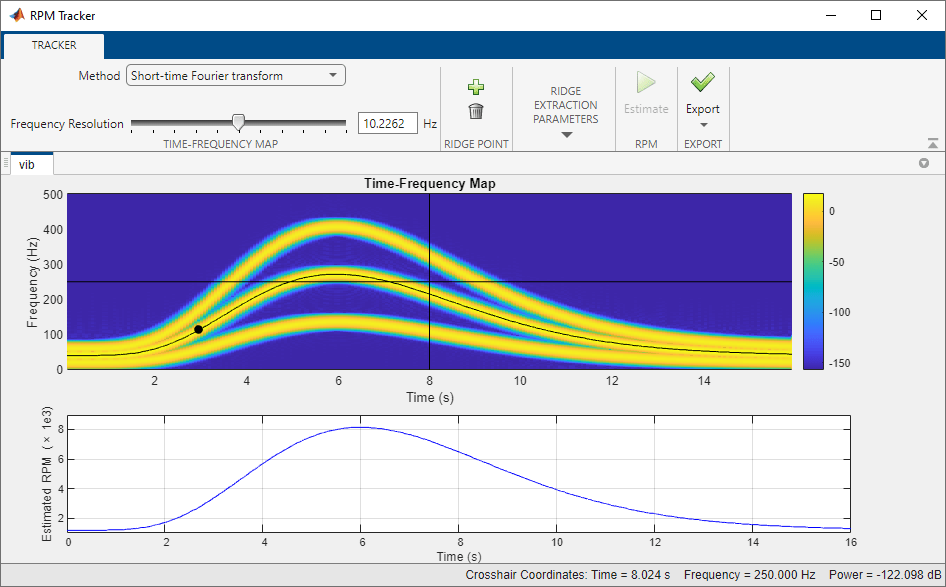Generate a signal that resembles the vibrations caused by revving a car engine. The signal is sampled at 1 kHz for 30 seconds and contains three harmonic components of orders 1, 2.4, and 3, with amplitudes 5, 4, and 0.5, respectively. Embed the signal in unit-variance white Gaussian noise and store it in a MATLAB® timetable. Multiply the instantaneous frequency by 60 to obtain an RPM profile. Plot the RPM profile.

```fs = 1000; t = (0:1/fs:30)'; fit = @(a,x) (t-x).^6.*exp(-(t-x)).*((t-x)>=0)*a'; fis = fit([0.4 1 0.6 1],[0 6 13 17]); phi = 2*pi*cumtrapz(t,fis); ol = [1 2.4 3]; amp = [5 4 0.5]'; vib = cos(phi.*ol)*amp + randn(size(t)); xt = timetable(seconds(t),vib); plot(t,fis*60)```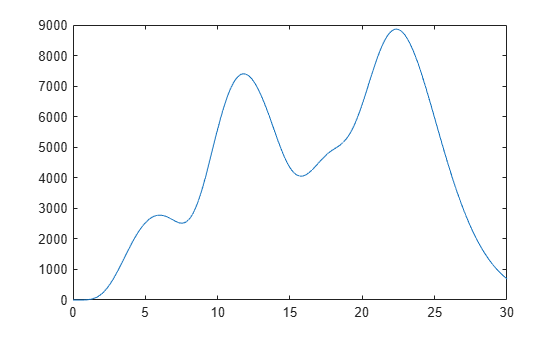Use the `rpmtrack` function to derive the RPM profile from the vibration signal. Use four points at 5 second intervals to specify the ridge corresponding to order 2.4. Display a summary of the output timetable.

```ndx = (5:5:20)*fs; order = ol(2); p = [t(ndx) order*fis(ndx)]; rpmest = rpmtrack(xt,order,p); summary(rpmest)```
```RowTimes: tout: 30001x1 duration Values: Min 0 sec Median 15 sec Max 30 sec TimeStep 0.001 sec Variables: rpm: 30001x1 double Values: Min 2.2204e-16 Median 4327.2 Max 8879.8 ```

Plot the reconstructed RPM profile and the points used in the reconstruction.

```hold on plot(seconds(rpmest.tout),rpmest.rpm,'.-') plot(t(ndx),fis(ndx)*60,'ok') hold off legend('Original','Reconstructed','Ridge points','Location','northwest')```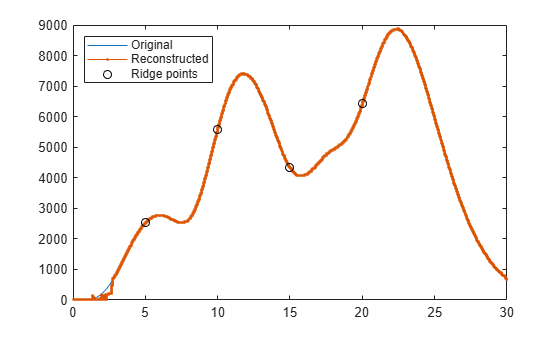Use the extracted RPM profile to generate the order-RPM map of the signal.

`rpmordermap(vib,fs,rpmest.rpm)`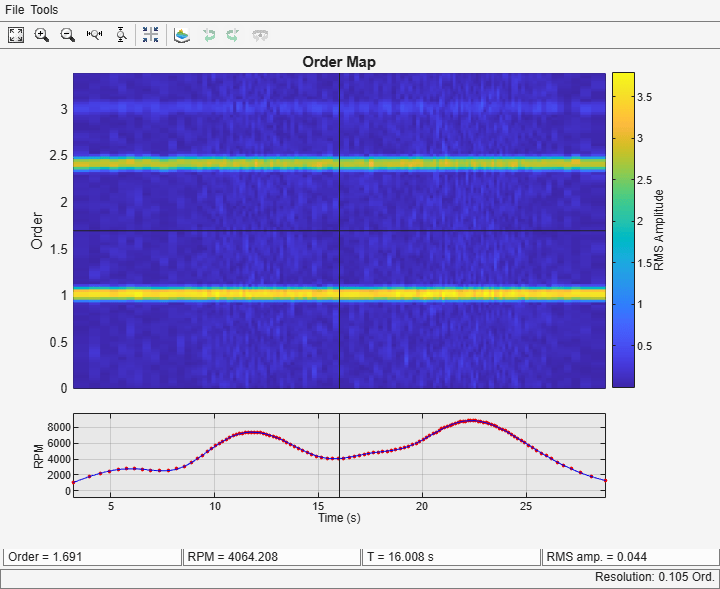Reconstruct and plot the time-domain waveforms that compose the signal. Zoom in on a time interval occurring after the transients have decayed.

```xrc = orderwaveform(vib,fs,rpmest.rpm,ol); figure plot(t,xrc) legend([repmat('Order = ',[3 1]) num2str(ol')]) xlim([5 20])```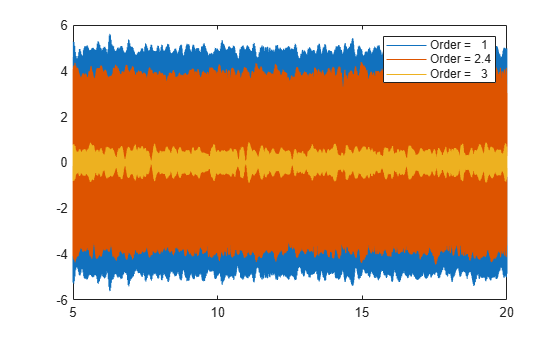Estimate the RPM profile of a fan blade as it slows down after switchoff.

An industrial roof fan spinning at 20,000 rpm is turned off. Air resistance (with a negligible contribution from bearing friction) causes the fan rotor to stop in approximately 6 seconds. A high-speed camera measures the x-coordinate of one of the fan blades at a rate of 1 kHz.

```fs = 1000; t = 0:1/fs:6-1/fs; rpm0 = 20000;```

Idealize the fan blade as a point mass circling the rotor center at a radius of 50 cm. The blade experiences a drag force proportional to speed, resulting in the following expression for the phase angle:

`$\varphi =2\pi {f}_{0}T\left(1-{e}^{-t/T}\right),$`

where ${\mathit{f}}_{0}$ is the initial frequency and $\mathit{T}=0.75$ second is the decay time.

```a = 0.5; f0 = rpm0/60; T = 0.75; phi = 2*pi*f0*T*(1-exp(-t/T));```

Compute and plot the x- and y-coordinates of the blade. Add white Gaussian noise of variance $0.{1}^{2}$.

```x = a*cos(phi) + randn(size(phi))/10; y = a*sin(phi) + randn(size(phi))/10; plot(t,x,t,y)```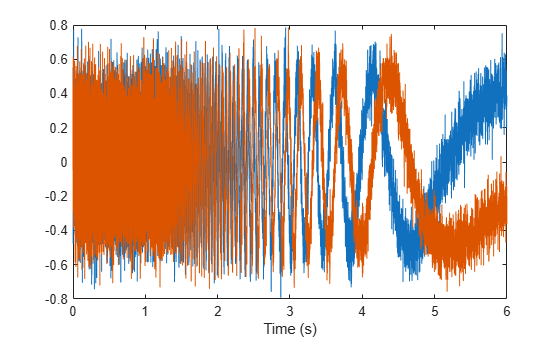Use the `rpmtrack` function to determine the RPM profile. Type

`rpmtrack(x,fs)`

at the command line to open the interactive figure.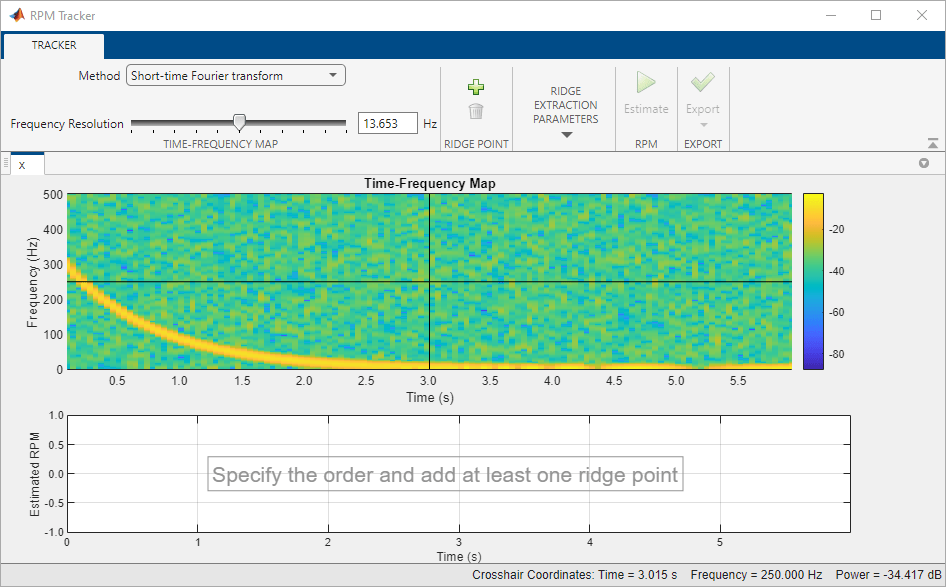Use the slider to adjust the frequency resolution of the time-frequency map to about 11 Hz. Assume that the signal component corresponds to order 1 and set the end time for ridge extraction to 3.0 seconds. Use the crosshair cursor in the time-frequency map and the Add button to add three points lying on the ridge. (Alternatively, double-click the cursor to add the points at the locations you choose.) Click Estimate to track and extract the RPM profile.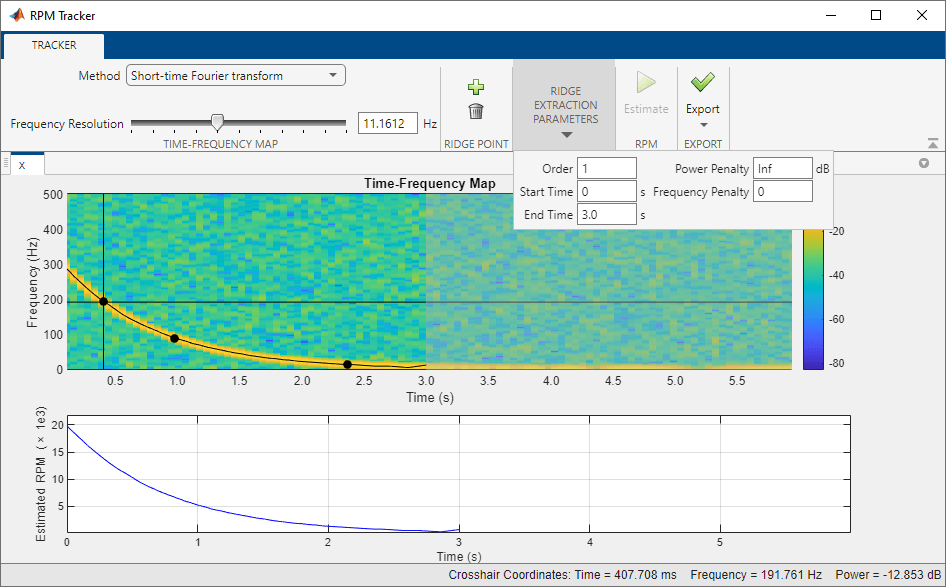Verify that the RPM profile decays exponentially. On the Export tab, click Export and select `Generate MATLAB Script`. The script appears in the Editor.

```% MATLAB Code from rpmtrack GUI % Generated by MATLAB 9.4 and Signal Processing Toolbox 8.0 % Generated on 18-Dec-2017 19:00:35 % Set sample rate fs = 1000.0000; % Set order of ridge of interest order = 1.0000; % Set ridge points on ridge of interest ridgePoints = [... 0.4501 179.8246;... 0.9944 88.5965;... 2.4161 11.4035]; % Estimate RPM [rpmOut,tOut] = rpmtrack(x,fs,order,ridgePoints,... 'Method','stft',... 'FrequencyResolution',11.1612,... 'PowerPenalty',Inf,... 'FrequencyPenalty',0.0000,... 'StartTime',0.0000,... 'EndTime',3.0000);```

Run the script. Display the RPM profile in a semilogarithmic plot.

```semilogy(tOut,rpmOut) ylim([500 20000])```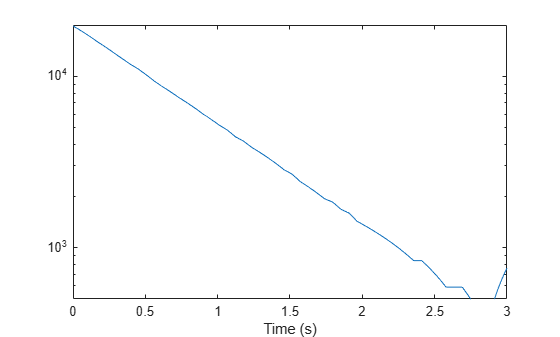## Input Arguments

collapse all

Input signal, specified as a vector.

Example: `cos(pi/4*(0:159))+randn(1,160)` specifies a noisy sinusoid sampled at 2π Hz.

Data Types: `single` | `double`

Sample rate, specified as a positive real scalar.

Data Types: `single` | `double`

Ridge order, specified as a positive real scalar.

Data Types: `single` | `double`

Ridge points, specified as a two-column matrix containing one time-frequency coordinate on each row. The coordinates describe points on the time-frequency map belonging to the order ridge of interest.

Data Types: `single` | `double`

Input timetable. `xt` must contain increasing, finite, and equally spaced row times of type `duration`. The timetable must contain only one numeric data vector with signal values.

If a timetable has missing or duplicate time points, you can fix it using the tips in Clean Timetable with Missing, Duplicate, or Nonuniform Times (MATLAB).

Example: `timetable(seconds(0:4)',randn(5,1))` specifies a random variable sampled at 1 Hz for 4 seconds.

Data Types: `single` | `double`

### Name-Value Pair Arguments

Specify optional comma-separated pairs of `Name,Value` arguments. `Name` is the argument name and `Value` is the corresponding value. `Name` must appear inside quotes. You can specify several name and value pair arguments in any order as `Name1,Value1,...,NameN,ValueN`.

Example: `'Method','fsst','PowerPenalty',10` specifies that the time-frequency map is estimated using the synchrosqueezed Fourier transform, allowing up to 10 decibels of power difference between adjacent points on a ridge.

Type of time-frequency map used in the estimation process, specified as the comma-separated pair consisting of `'Method'` and either `'stft'` or `'fsst'`.

• `'stft'` — Use the short-time Fourier transform to compute a power spectrogram time-frequency map. See `pspectrum` for more details about the short-time Fourier transform.

• `'fsst'` — Use the synchrosqueezed Fourier transform to compute a time-frequency map. See `fsst` for more details about the synchrosqueezed Fourier transform.

Frequency resolution bandwidth used to compute the time-frequency map, specified as the comma-separated pair consisting of `'FrequencyResolution'` and a numeric scalar expressed in Hz.

Data Types: `single` | `double`

Maximum difference in power between adjacent ridge points, specified as the comma-separated pair consisting of `'PowerPenalty'` and a numeric scalar expressed in dB.

Use this parameter to ensure that the ridge-extraction algorithm of `rpmtrack` finds the correct ridge for the corresponding order. `'PowerPenalty'` is useful when the order ridge of interest crosses other ridges or is very close in frequency to other ridges, but has a different power level.

Data Types: `single` | `double`

Penalty in coarse ridge extraction, specified as the comma-separated pair consisting of `'FrequencyPenalty'` and a nonnegative scalar.

Use this parameter to ensure that the ridge-extraction algorithm of `rpmtrack` avoids large jumps that could make the ridge estimate move to an incorrect time-frequency location. `'FrequencyPenalty'` is useful when you want to differentiate order ridges that cross or are closely spaced in frequency.

Data Types: `single` | `double`

Start time for RPM profile estimation, specified as the comma-separated pair consisting of `'StartTime'` and a numeric or `duration` scalar.

Data Types: `single` | `double` | `duration`

End time for RPM profile estimation, specified as the comma-separated pair consisting of `'EndTime'` and a numeric or `duration` scalar.

Data Types: `single` | `double` | `duration`

## Output Arguments

collapse all

Rotational speed estimate, returned as a vector expressed in revolutions per minute.

If the input to `rpmtrack` is a timetable, then `rpm` is also a timetable with a single variable labeled `rpm`. The row times of the timetable are labeled `tout` and are of type `duration`.

Time values at which the RPM profile is estimated, returned as a vector.

## Algorithms

`rpmtrack` uses a two-step (coarse-fine) estimation method:

1. Compute a time-frequency map of `x` and extract a time-frequency ridge based on a specified set of points on the ridge, `p`, the `order` corresponding to that ridge, and the optional penalty parameters `'PowerPenalty'` and `'FrequencyPenalty'`. The extracted ridge provides a coarse estimate of the RPM profile.

2. Compute the order waveform corresponding to the extracted ridge using a Vold-Kalman filter and calculate a new time-frequency map from this waveform. The isolated order ridge from the new time-frequency map provides a fine estimate of the RPM profile.

 Urbanek, Jacek, Tomasz Barszcz, and Jerome Antoni. "A Two-Step Procedure for Estimation of Instantaneous Rotational Speed with Large Fluctuations." Mechanical Systems and Signal Processing. Vol. 38, 2013, pp. 96–102.